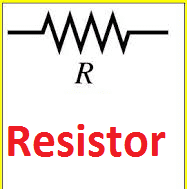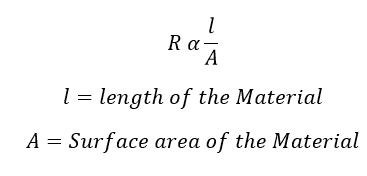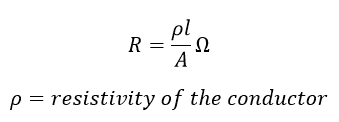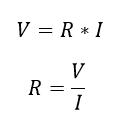# Definition of Resistance

The fundamental properties of a matter or substances which oppose the flow of electron is called electrical resistance. The resistor is a component which opposes the flow of electron(current). Resistance is a measured value of a resistor.For better understanding, apply a voltage across a conductor, just observe the conductor carefully, you can see the variation in the current flowing through the conductor. The current in one end is not equal to another end, that you can see. This is because current carrying capacity of all material is not equal. Here the conductor allows, the flow of charges but conductor’s resistance opposes the flow electron. Also, the value of current is depending upon the numbers of electrons crossing through the conductor and the number of free electrons available in the material structure.

When we apply a voltage across a material, the electron tries to move across the conductor within the material’s structure. If the electrons are bound tightly, then it will not be easy to pull them the electron freely. So there The first is that the ease with, which electrons are able to move within the structure of the material. In other materials, there are very many free electrons drifting randomly around the lattice. It is these materials that allow a current to flow more easily.

Consider a substance has many free electron means; the amount of current is more for the same applied Voltage. The current through the material is not only depending upon free electron, it depends upon electron the path of the material. In addition to that, every electron has to collide randomly with other atoms and electrons in numbers of times during its traveling. So, every substance has a property to resist current through it and this property is known as electric resistance.

The Substances, the higher number of the free electron has the lower resistance which uses an electrical conductor. Few examples: Copper, aluminum, gold, iron etc.

The substances have the zero number of free electron, which has higher resistance value. The same substances use as an insulator. Few examples of insulator Plastic, mica, porcelain, varnish, rubber etc.

## Unit of Resistance:

The Sl unit of resistance is OHM, this is honored by 19th-century German physicist George Simon Ohm.

Their circuit resistance is Normally depending upon Four important factor.

1) The surface area of the Material

2) length of Material

3) MOC (Material of construction)

Here the Substances resistance is directly proportional to the length of the conductor and indirection proportional to surface area of the conductor.The length of the material increases the resistance of the material also increase and the surface is of the conductor increase the resistance of the same material will get decreased. To get the exact value the proportionality constant resistivity will be added with the expression.The resistivity will be varied according to the material,

Resistivity is the third variable which affects the resistance of the material. Which is depending upon the material is made of. All the material does not have the equal conducting ability, some of the materials are having less resistance to flow of current. The conducting ability of a material is often indicated by its resistivity. The resistivity of a material is dependent upon the material’s atomic structure and its temperature.

 S.No Material Resistivity (ohm•meter) Conductor Silver 1.59 x 10-8 Copper 1.7 x 10-8 Gold 2.2 x 10-8 Aluminum 2.8 x 10-8 Tungsten 5.6 x 10-8 Iron 10 x 10-8 Platinum 11 x 10-8 Lead 22 x 10-8 Nichrome 150 x 10-8 Carbon 3.5 x 10-5 Semi Condutor Germanium 0.046 Silicon 2300 Insulator Polystyrene 107 – 1011 Polyethylene 108 – 109 Glass 1010 – 1014

Refer, Above table Various resistivity of the material.

## Conclusion:

Resistance is the measure of the current opposing capacity of the material. R is the symbolic representation The circuit element which does this perfectly is called the “Resistor”.

Resistance cannot be negative and it is always positive. Resistance also says about the relationship between the current and voltage.

From Ohm’s law### The definition of One Ohm:

A conductor is said to have the resistance of one ohm if it permits one ampere of current flow through it when one volt applied across its temperature.

The conductor is defined according to its resistance value.

Low resistance material => Good Conductor

High resistance material => Bad conductor

Low resistance, for example, 1Ω or less implies that the circuit is a good conductor made from materials such as copper, aluminum or carbon while a high resistance, 1MΩ or more implies the circuit is a bad conductor made from insulating materials such as glass, porcelain or plastic.

Resistance is pure passive components and is not affected by the frequency with the AC impedance of a resistance being equal to its DC resistance and as a result, cannot be negative.